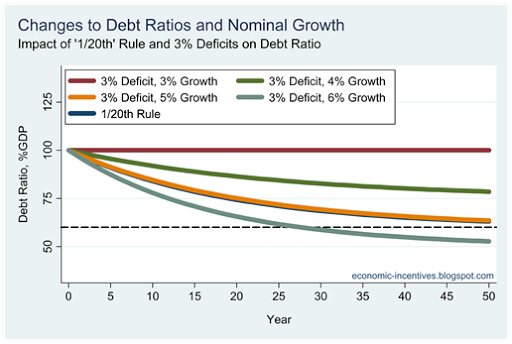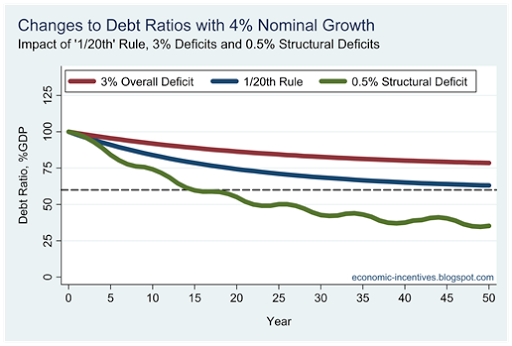## Wednesday, May 9, 2012

### Debt and Deficits in EU Fiscal Rules

The emphasis of the original Stability and Growth Pact was entirely on insuring that deficits did not exceed the 3% of GDP threshold, and it was taken that low deficits and nominal growth would do the work of bringing down the high debt ratios.

The focus on deficits has been evident since the original entry criteria laid out in the Maastricht Treaty.  The 1992 Maastricht Treaty provided the reference values for the annual deficit (3% of GDP) and the stock of debt (60% of GDP).  However, greater emphasis was placed on the deficit rule.

To gain entry to the euro is was necessary that a country’s deficit was “close to the reference value”, but for the level of debt all that was necessary was that the debt ratio was “sufficiently diminishing and approaching the reference value at a satisfactory pace”.

No numerical benchmark was provided to define satisfactory pace.  Even though they had debt ratios in excess of 100% of GDP both Belgium and Italy were cleared for entry into the euro in 1999 as the rate of reduction in the debt ratio was deemed ‘satisfactory’. Greece was admitted in 2001 with a debt ratio that was also above 100% of GDP, but it was actually increasing rather than falling.

The performance of annual deficits relative to the Maastricht criteria was much better and all the original 11 members had deficits of less than 3% of GDP in 1999.  The ‘close to’ requirement under the deficit rule was much less accommodating than the ‘sufficiently diminishing’ flexibility allowed under the debt rule.  Of course, in 2001 Greece was allowed into the single currency with a deficit of 4.5% of GDP and it had been 1980 since it last had a deficit below the 3% of GDP reference value.

As the launch of the euro approached in the mid-nineties the EU put together the framework that would oversee fiscal outcomes in the EU.  This saw the introduction of the Stability and Growth Pact.  This took the reference values from the Maastricht Treaty and incorporation them into two EU Council Regulations; the ‘preventative’ arm and the ‘corrective’ arm.

It was in the preventative arm that countries agreed to “the objective of sound budgetary positions close to balance or in surplus”.  It is 15 years since Ireland first committed to a balanced-budget rule.

The corrective arm of the Stability and Growth Pact set out what was to happen if a country exceeded the reference values, but the emphasis was entirely on the annual deficit.  If a country’s annual deficit exceeded the 3% of GDP threshold, that country would be put in an Excessive Deficit Procedure (EDP) and be required to try and bring its deficit until the 3% of GDP benchmark.  If the country failed to introduce measures to try and curb the deficit it would face fines of up to 0.1% of GDP.

Although a reference value was set for the level of debt there were no fines or sanctions for countries that exceeded the 60% of GDP threshold.  The emphasis of the corrective arm was entirely on the annual deficit.  The view was that if deficits were sufficiently curtailed that low deficits in conjunction with economic growth would bring the ratio down.

The Maastricht criteria was internally consistent with a world in which nominal GDP growth was 5%.  In such a world average deficits of 3% of GDP would see the debt-t0-GDP ratio converge on 60% regardless of the initial starting position.  The view was that the emphasis on deficits was sufficient as the debt ratio would improve with reductions in deficits.

The reality did not fit with the view.  From 2000 to 2008, Italy had an average nominal growth rate of 3.2%, while at the same time it ran average deficits of 2.9% of GDP.  In such an environment the debt ratio will not change by much and Italy’s 2008 debt ratio of 106% was not much different from the debt level it carried into the euro in 1999.

Under the rules of the Stability and Growth Pact there was little that could be done against such a continued exceeding of the reference value for government debt as the corrective arm was framed entirely in terms of the annual deficit.  Italy needed nominal growth to close to 5% to bring the debt ratio down but this did not materialise.

Greece actually exceeded the assumed nominal growth rate and between 2000 and 2008, nominal GDP growth in Greece averaged around 7% per annum.  The problem was that Greece has average deficits of just over 6% of GDP.  Although Greece had the nominal growth to allow the debt ratio to fall, this space was filled by additional borrowing required because of the large deficits.

So why didn’t the Excessive Deficit Procedure (EDP) as outlined in the 1997 Stability and Growth Pact and require Greece to bring the deficit below the 3% of GDP reference value and create the fiscal space for the debt ratio to fall?  Greece was put into an EDP in 2004 but was allowed to exit the EDP in 2007 when the Council ruled that the Greek deficit would fall below the 3% of GDP reference value.  This was not true as Greece was hiding the true extent of the deficits.  The excessive deficit was not corrected and the 2007 deficit was actually more the twice the allowable level at 6.5% of GDP.

As part of the ‘six pack’ agreed last year a numerical reduction was agreed to provide a benchmark for the required falls in the debt ratio.  As John McHale has shown that ‘1/20th’ rule is actually the equivalent of the 3% deficit rule in a situation where nominal growth is 5%.  The debt brake rule takes the expectation of the Maastricht Treaty and formalises it.  Here is a graph that illustrates this point.This shows what happens to the debt ratio in a country that rules deficits of 3% of GDP and experiences different nominal growth rates.  The starting point is a country with a debt ratio equal to 100% of GDP.

With 3% nominal growth, the debt ratio will not change as the growth in the numerator (debt) is the same as the growth in the denominator (GDP).  With deficits of 3% of GDP it takes nominal growth of greater than 3% to bring the ratio down.  With 4% growth the ratio falls, albeit very slowly and with 5% growth the ratio can be seen to converge on the 60% of GDP reference value.  If growth is 6% the ratio will decline more rapidly and converge on 50% of GDP.  At higher growth rates the rate of decline increases and the level the debt converges on gets lower (provided the country continues to run deficits of 3% of GDP).

The graph also includes the debt reduction requirement of the 1/20th rule.  This sets a numerical benchmark for the reduction in the debt ratio, and in the strictest sense is independent of the debt ratio.  The line for the 1/20th rule can hardly be seen.  This is because it is almost completely covered by the line represented 3% deficits and 5% nominal GDP growth (the assumptions of the Maastricht Treaty).  What is being required in the debt brake of 2011 is no more than was expected under the Maastricht criteria in 1992.

The rules are virtually equivalent in the case of 5% nominal GDP growth.  With 6% growth the debt-brake will be non-binding as the requirements of the 3% deficit rule will exceed it. At growth rates lower than 5% the debt brake will be binding and will force reductions in the debt ratio that are larger than those that can be achieved by running 3% deficits.

Of course, it is important to note that 3% of GDP is the limit for deficits and not the target.  Since 1997, EU countries have committed to running budgets “close to balance or in surplus”.  In 2005, the balanced-budget rule was restated in terms of the structural deficit and a limit of –0.5% of GDP was placed in the structural balance via country’s Medium Term Budgetary Objective.  This will be more restrictive than the debt brake rule.

Here is a graph that shows the changes in the debt ratio with 4% nominal growth under the 3% of GDP deficit limit, the ‘1/20th’ debt brake and the 0.5% of GDP structural deficit limit.  For the latter I have assumed that the cyclical element of the budget balance as a percent of GDP follows the pattern –2%, –1%, 0%, +1%, 2%, +1%, 0%, –1% and so on, and allowed a structural deficit of 1.0% of GDP once the debt ratio falls below the 60% threshold.It can be seen that the structural deficit rule is far more restrictive and, in the example here with 4% nominal growth, will force the debt ratio to converge on a level equal to 25% of GDP.  This is well below the 60% of GDP reference value but that appears to be the intention.